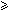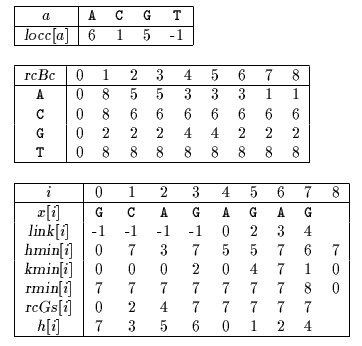Name
• String
• Substring
• Search
Edit

The character comparisons are done using a specific order given by a table h.

For each integer i such thatim we define two disjoint sets:Pos(i)={k :  0ki and x[i] = x[i-k]}Neg(i)={k :  0ki and x[i]x[i-k]}

Forkm, let hmin[k] be the minimum integersuch thatk-1 and k not in Neg(i) for all i such that< im-1.

Form-1, let kmin[] be the minimum integer k such that hmin[k]=k if any such k exists and kmin[]=0 otherwise.

Form-1, let rmin[] be the minimum integer k such that r >and hmin[r]=r-1.

The value of h is set to m-1. After that we choose in increasing order of kmin[], all the indexes h, ... , h[d] such that kmin[h[i]]0 and we set rcGs[i] to kmin[h[i]] forid. Then we choose the indexes h[d+1], ... , h[m-1] in increasing order and we set rcGs[i] to rmin[h[i]] for d < i < m.

The value of rcGs[m] is set to the period of x.

The table rcBc is defined as follows: rcBc[as]=min{k :  (k=m or x[m-k-1]=a) and (k > m-s-1 or x[m-k-s-1]=x[m-s-1])} To compute the table rcBc we define: for each c inlocc[c] is the index of the rightmost occurrence of c in x[0 .. m-2] (locc[c] is set to -1 if c does not occur in x[0 .. m-2]).

A table link is used to link downward all the occurrences of each pattern character.

The preprocessing phase can be performed in O(m2) time and O(m) space complexity. The searching phase is in O(n) time complexity.

Main features:

• refinement of the Boyer-Moore algorithm;
• partitions the set of pattern positions into two disjoint subsets;
• preprocessing phase in O(m2) time and O(m) space;
• searching phase in O(n) time complexity;
• 2n text character comparisons in the worst case.

Example:

Preprocessing phaseTables used by Reverse Colussi algorithm

Searching phase

 G C A T C G C A G A G A G T A T A C A G T A C G 1 G C A G A G A G

Shift by: 1 (rcBc[A])

 G C A T C G C A G A G A G T A T A C A G T A C G 2 1 G C A G A G A G

Shift by: 2 (rcGs)

 G C A T C G C A G A G A G T A T A C A G T A C G 2 1 G C A G A G A G

Shift by: 2 (rcGs)

 G C A T C G C A G A G A G T A T A C A G T A C G 5 6 7 2 8 3 4 1 G C A G A G A G

Shift by: 7 (rcGs)

 G C A T C G C A G A G A G T A T A C A G T A C G 2 1 G C A G A G A G

Shift by: 2 (rcGs)

 G C A T C G C A G A G A G T A T A C A G T A C G 1 G C A G A G A G

Shift by: 5 (rcBc[A])

The Reverse Colussi algorithm performs 16 character comparisons on the example.

C